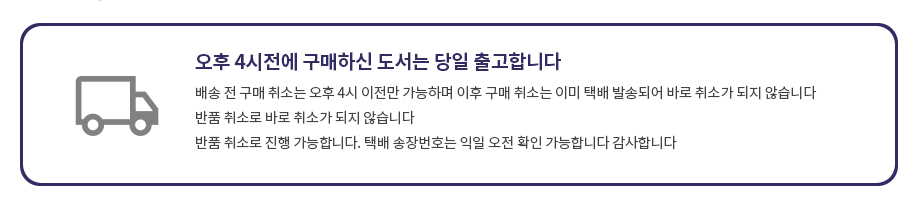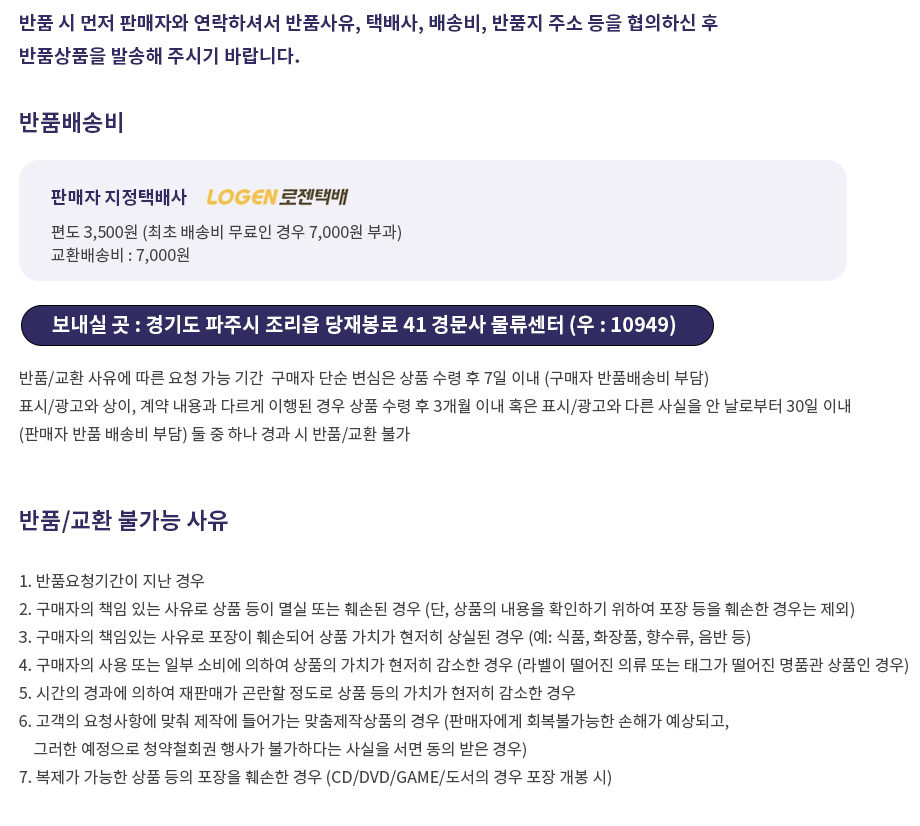## 로그인이필요합니다## 도서를 검색해 주세요.

원하시는 결과가 없으시면 문의 주시거나 다른 검색어를 입력해보세요.

•상품상세

## Applied Statistics and Probability for Engineers, 3rd - Student Workbook with Solustions 요약정보 및 구매

상품 선택옵션 0 개, 추가옵션 0 개

사용후기 0 개
지은이 Montgomery Runger 2004-01-20 9780471426820 구매가능 35,000원 0점 주문시 결제

### 선택된 옵션

• Applied Statistics and Probability for Engineers, 3rd - Student Workbook with Solustions
+0원

## 관련상품

• This best-selling engineering statistics text provides a practical approach that is more oriented to engineering and the chemical and physical sciences than many similar texts. It's packed with unique problem sets that reflect realistic situations engineers will encounter in their working lives. Each copy of the book includes an e-Text on CD - that is a complete electronic version of book. This e-Text features enlarged figures, worked-out solutions, links to data sets for problems solved with a computer, multiple links between glossary terms and text sections for quick and easy reference, and a wealth of additional material to create a dynamic study environment for students. Suitable for a one- or two-term Jr/Sr course in probability and statistics for all engineering majors.
• Chapter 1. The Role of Statistics in Engineering. Chapter 2. Probability. Chapter 3. Discrete Random Variables and Probability Distributions. Chapter 4. Continuous Random Variables and Probability Distributions. Chapter 5. Joint Probability Distributions. Chapter 6. Descriptive Statistics. Chapter 7. Sampling Distributions and Point Estimation of Parameters. Chapter 8. Statistical Intervals for a Single Sample. Chapter 9. Tests of Hypotheses for a Single Sample. Chapter 10. Statistical Inference for Two Samples. Chapter 11. Simple Linear Regression and Correlation. Chapter 12. Multiple Linear Regression. Chapter 13. Design and Analysis of Single factor Experiments: The Analysis of Variance. Chapter 14. Design of Experiments with Several Factors. Chapter 15. Statistical Quality Control. Appendices. Appendix A. Statistical Tables and Charts. Appendix B. Answers to Selected Exercises. Appendix C. Bibliography. Glossary. Index. Index of Applications in Example and Exercises, Continued.
• 학습자료

등록된 학습자료가 없습니다.

정오표

등록된 정오표가 없습니다.

사용후기가 없습니다.

상품문의가 없습니다.

• ## 배송/교환정보

### 배송정보### 교환/반품 정보### 선택된 옵션

• Applied Statistics and Probability for Engineers, 3rd - Student Workbook with Solustions
+0원